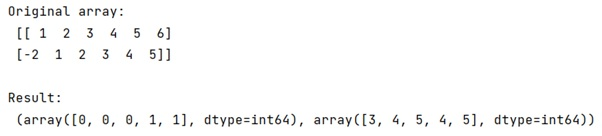# Why the output of numpy.where(condition) is not an array, but a tuple of arrays?

Learn, why the output of numpy.where(condition) is not an array, but a tuple of arrays?
Submitted by Pranit Sharma, on February 21, 2023

NumPy is an abbreviated form of Numerical Python. It is used for different types of scientific operations in python. Numpy is a vast library in python which is used for almost every kind of scientific or mathematical operation. It is itself an array which is a collection of various methods and functions for processing the arrays.

## Output of numpy.where(condition)

Basically, numpy.where do have 2 'operational modes', first one returns the indices, where the condition is True and if optional parameters x and y are present (same shape as condition, or broadcastable to such shape!), it will return values from x when the condition is True otherwise from y. So, this makes where more versatile and enables it to be used more often.

It returns a tuple of length equal to the dimension of the numpy ndarray on which it is called (in other words ndim) and each item of the tuple is a numpy ndarray of indices of all those values in the initial ndarray for which the condition is True.

When it returns a tuple, each element of the tuple refers to a dimension. We can understand with the help of the following example,

## Python code to demonstrate why the output of numpy.where(condition) is not an array, but a tuple of arrays

```# Import numpy
import numpy as np

# Creating a numpy array
arr = np.array([
[1, 2, 3, 4, 5, 6],
[-2, 1, 2, 3, 4, 5]])

# Display original array
print("Original array:\n",arr,"\n")

# using where
res = np.where(arr > 3)

# Display result
print("Result:\n",res)
```

Output:As we can see, the first element of the tuple refers to the first dimension of relevant elements; the second element refers to the second dimension.